# DP-选课（记忆化搜索）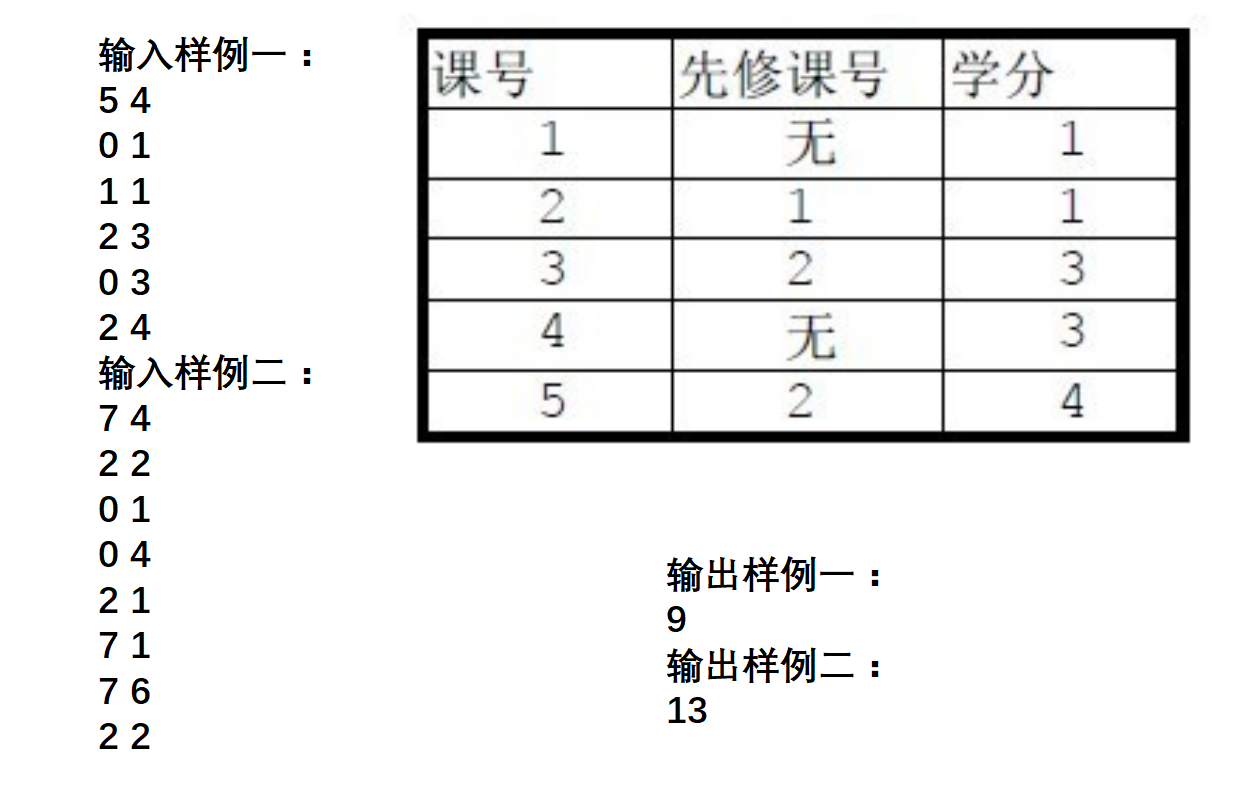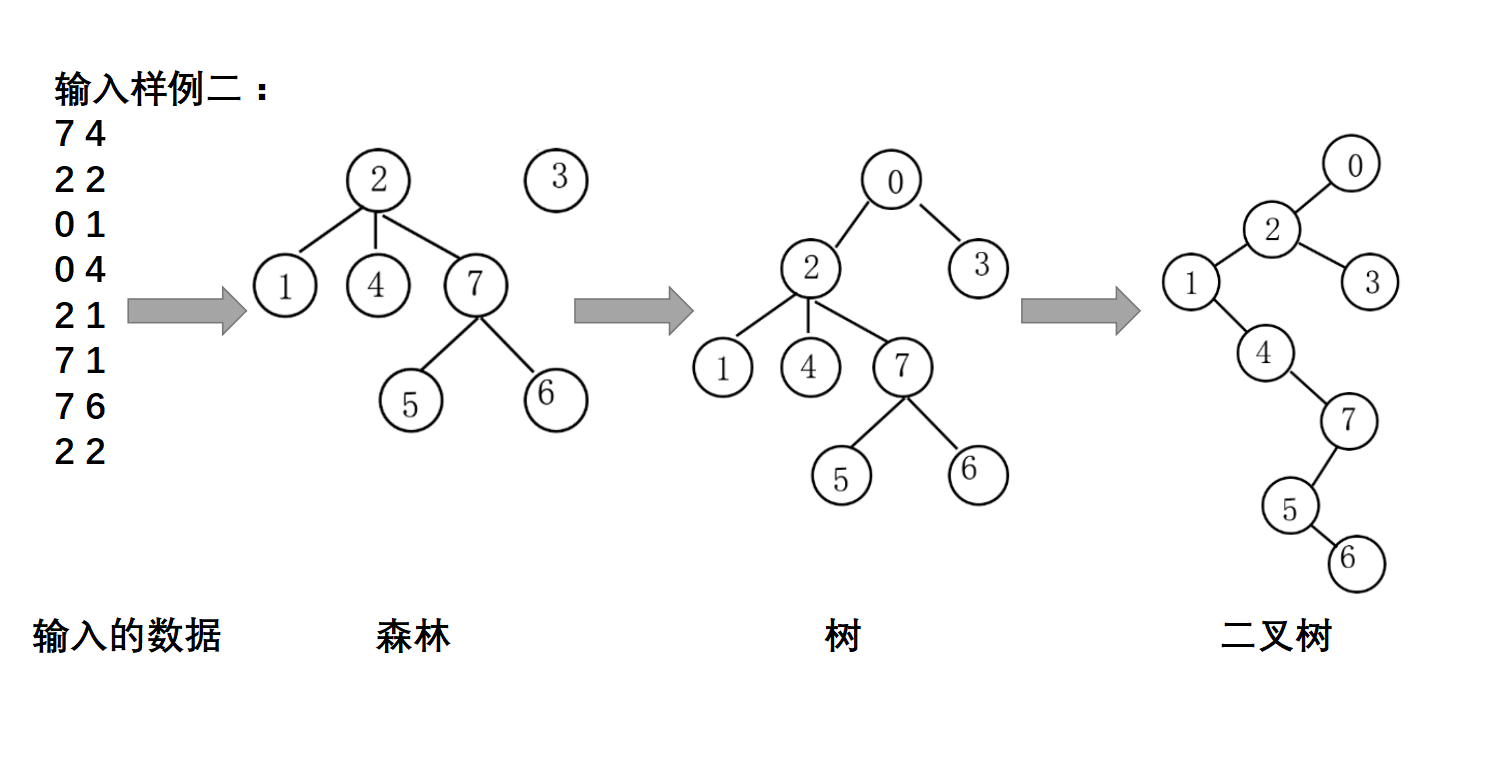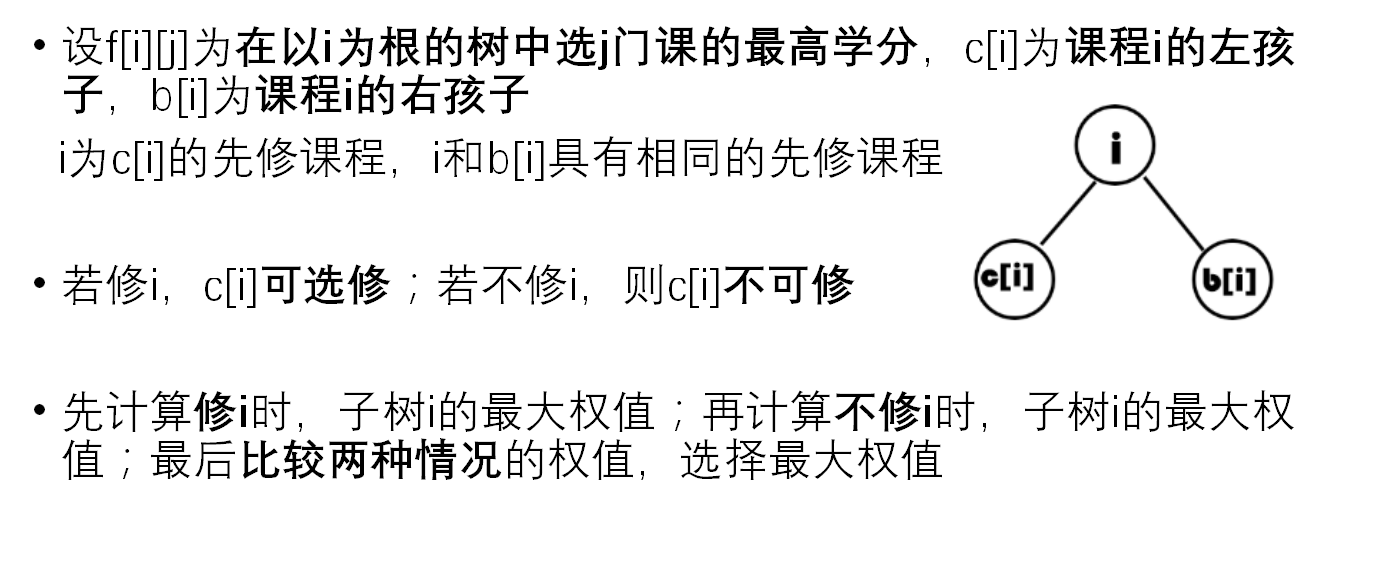f[root][num]=max(
//不修root：在树b[root]中选取num项课程
f[b[root]][num],
//修root：在树c[root] 和树b[root] 中 共选取num-1项课程
score[root]+f[c[root]][k]+f[b[root]][num-k-1]

#include<iostream>
#define max(a,b) (a>b?a:b)
using namespace std;
//c[i]:i的child(左孩子)；b[i]:i的brother(右孩子)；score[i]:课程i的学分
//f[i][j]:在以i为根的树中选择j门课程的最高学分
int score,n,m,c,b,f;
void init();//输入数据并转化为孩子兄弟表示法的二叉树
int dfs(int root,int num);//使用记忆化搜索得出以root为根选num门可的最高学分

int main()
{
init();
cout<<dfs(c,m)<<endl;
return 0;
}
void init()
{
int t;
cin>>n>>m;
for(int i=1;i<=n;i++) {
//当前循环中，i为课程号，t为i的先修课，score为i的学分
cin>>t>>score[i];
//t的后修课程c[t]与i具有相同的先修课程t
b[i]=c[t];
c[t]=i;
}
}

//root:当前层的根编号，num:当前子树中应选课程数
int dfs(int root,int num)
{
if(!root||!num) return 0;
if(f[root][num]) return f[root][num];
//记录以root为根的树中，修课程root且选择num门课的最高学分
for(int k=0;k<num;k++)
f[root][num]=max(f[root][num],dfs(c[root],k)+dfs(b[root],num-k-1)+score[root]);
//比较 修或不修课程root所能达到的最高学分
return f[root][num]=max(f[root][num],dfs(b[root],num));
}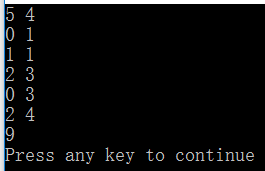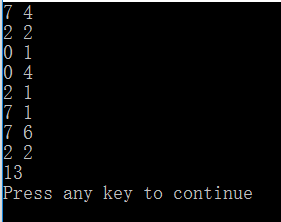int dfs(int root,int num)
{
if(!root||!num) return 0;
if(f[root][num]) return f[root][num];
for(int k=0;k<num;k++)
f[root][num]=max(f[root][num],dfs(c[root],k)+dfs(b[root],num-k-1)+score[root]);
return f[root][num]=max(f[root][num],dfs(b[root],num));
}